Function Repository Resource:

Compute the radius of aberrancy of a plane curve

Contributed by: Jan Mangaldan
 ResourceFunction["RadiusOfAberrancy"][c,t] computes the radius of aberrancy of the plane curve c parametrized by variable t.

## Details

The radius of aberrancy of a plane curve at a given point is defined as the signed distance between the point and its corresponding center of aberrancy.

## Examples

### Basic Examples (3)

Compute the radius of aberrancy for a curve:

 In:=Out=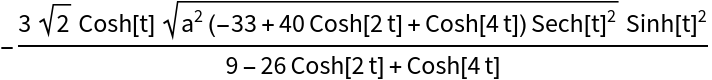Compute the radius of aberrancy for several curves:

 In:=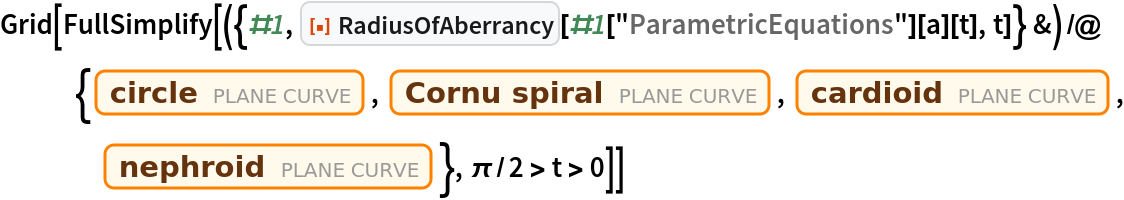Out=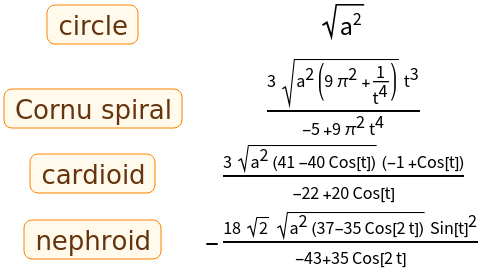Compute the radius of aberrancy of a curve in polar coordinates:

 In:=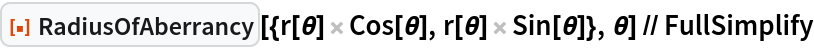Out=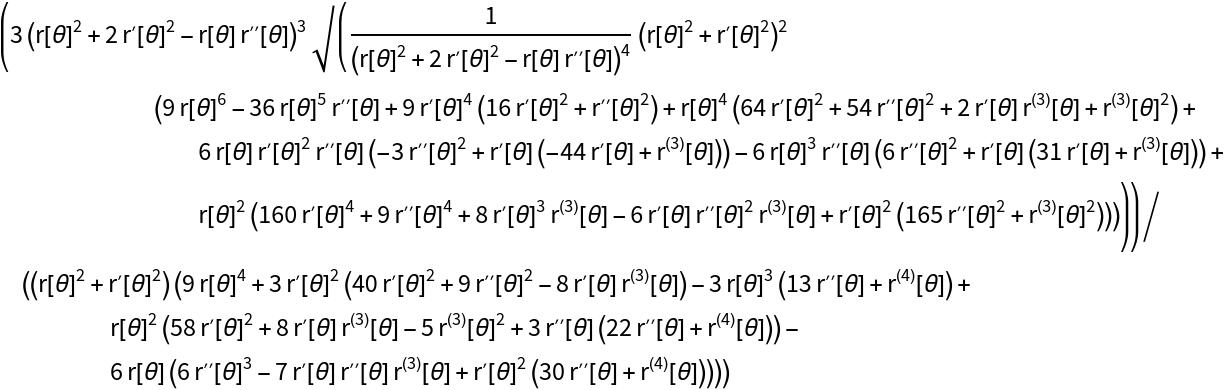### Properties and Relations (3)

The radius of aberrancy of a curve at a given point is the signed distance between that point and its corresponding center of aberrancy:

 In:=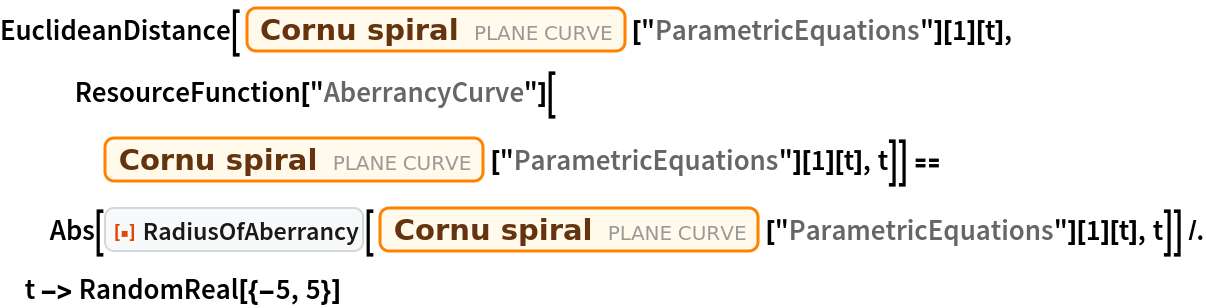Out=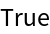The radius of aberrancy of a curve involves third and fourth derivatives:

 In:=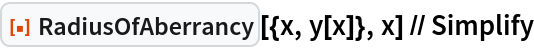Out=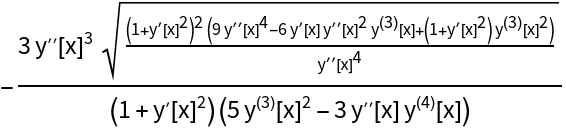The radius of aberrancy is related to the aberrancy and the tangential angle:

 In:=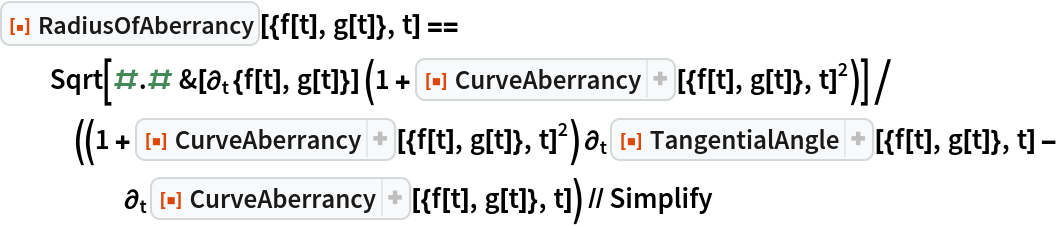Out=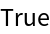## Version History

• 1.0.0 – 04 March 2021